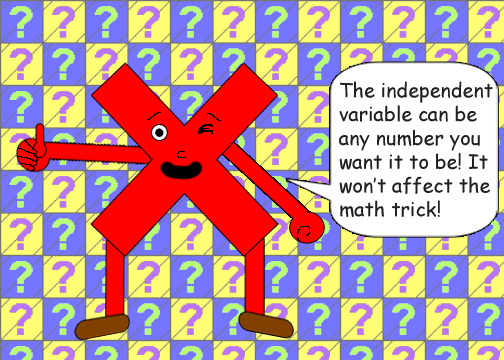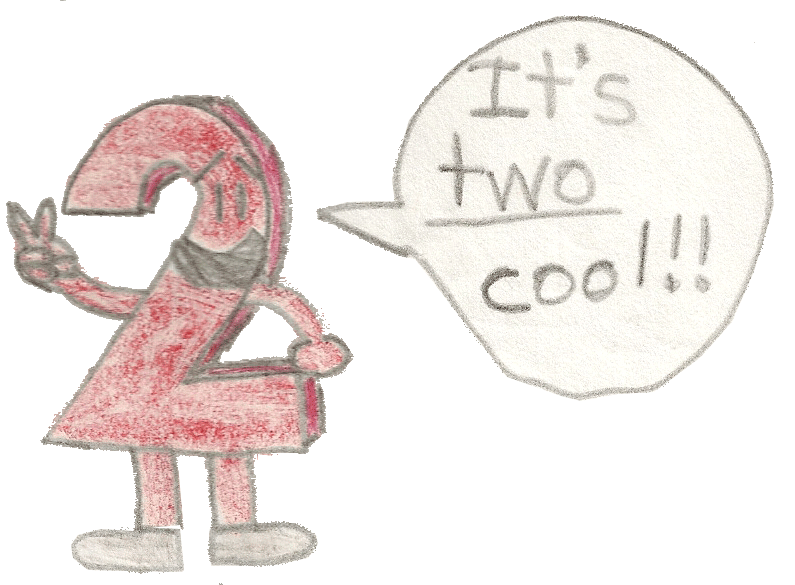# Square Root Surprise

## Examples:

-3 + √(2) - (√(2) - 1) = -2

1 + √(2) - (√(2) - 1) = 2

3 + √(2) - (√(2) - 1) = 4

½ + √(2) - (√(2) - 1) = 1 + ½

√(3) + √(2) - (√(2) - 1) = √(3) + 1

0 + √(2) - (√(2) - 1) = 1Miss Zero Digit representing the number zero(0) again!

You can also plug an imaginary or complex number into X!i + √(2) - (√(2) - 1) = 1 + i

-i + √(2) - (√(2) - 1) = 1 - i

(1 + i) + √(2) - (√(2) - 1) = 2 + i

(1 - i) + √(2) - (√(2) - 1) = 2 - i

(-2 + i) + √(2) - (√(2) - 1) = -1 + i

(-2 - i) + √(2) - (√(2) - 1) = -1 - i

What I just noticed with the complex numbers is that if the independent complex number has a plus between the real & imaginary values, then so will the dependent complex number! (The independent one is to the left of the equal sign.) That goes double for a minus!

## None of these examples look like they're correct, do they? But here's why all of this is true:

1 + (√(2) - 1) = √(2)

The binomial in parentheses: (√(2) - 1) is the difference between the square root of 2 & 1. Adding the difference between two numbers to the smaller one will always give you the bigger one as the sum! Surprise!